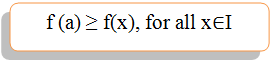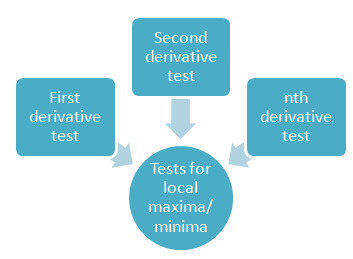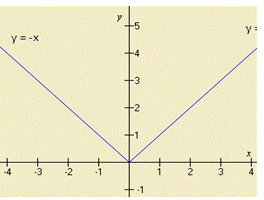Click to Chat

1800-1023-196

+91-120-4616500

CART 0

• 0

MY CART (5)

Use Coupon: CART20 and get 20% off on all online Study Material

ITEM
DETAILS
MRP
DISCOUNT
FINAL PRICE
Total Price: Rs.

There are no items in this cart.
Continue Shopping```Exploring the World of Maxima and Minima for IIT-JEE!!

Table of Content

The various topics that form a part of maxima and minima include

Local Maxima and Minima

Global Maxima and Minima

What are the various ways of testing the local maximum and minimum of a function?

Some Interesting Facts

Tips to Study Maxima and Minima?

Practical Applications of Maxima and MinimaOne of the major applications of derivatives is determination of the maximum and minimum values of a function. We know that the derivative provides the information about the gradient of the graph of a function which can be used to identify the points where the gradient is zero. These points are usually related to the largest and the smallest values of the function.

The maximum and minimum values of a function are together termed as extrema. Pierre de Fermat was one of the mathematicians to propose a general technique for finding the maxima & minima.Here, we shall throw some light on some interesting facts about maxima and minima including its sub-topics. And that’s not all…. we shall go into the intricacies and discuss the tips to master this topic and the practical applications of maxima and minima in real life as well.

The various topics that form a part of maxima and minima include

Stationary points

Turning points

Local maxima and  minima

Ways to identify local maxima and minima

Global maxima and minima

We shall give a brief outline of these topics in addition to the tips to master them. Those willing to go into the intricacies can refer the study material page on maxima and minima.

Local Maxima and Minima

Mathematically, we can define the local maxima of a function f to be a point ‘a’ such that f(a) > f(a-h) and f(a) > f(a+h), where h > 0 is a small quantity.

Similarly, a function f(x) is said to have a local minimum at the point ‘a’ if the value of the function at the point x = a is less than the value at the neighboring points.Global Maxima and Minima

Global maxima or minima of f(x) in [a, b] refers to the greatest or least value of f(x) in [a, b]. Mathematically, it can be explained as:

The function f(x) has a global maximum at the point ‘a’ in the interval I ifSimilarly, f(x) has a global minimum at the point ‘a’ if f (a) ≤f (x), for all x ∈ I.From the figure given below, it becomes clear that while global maxima denotes the maxima of the function in the entire interval, local maxima denotes the poitn at which the value of the function is maximum as compare to the neighbouring points.What are the various ways of testing the local maximum and minimum of a function?

Generally speaking, there are three ways to test a function for local maxima/ minima:Is the topic on maxima and minima important part of IIT JEE preparation? If yes, then which books should be referred for it?Maxima & Minima is an extremely important topic of differential calculus. One cannot dare to leave this topic as it often fetches good number of questions in JEE. The differential calculus portion accounts for 13% of the JEE screening and maxima & minima is an important component of application of derivatives. Some of the books which are considered to be best for preparation of calculus portion are:

Arihant Differential Calculus and

Das Gupta Calculus.

These books not only explain the concepts in detail but also contain various solved and unsolved examples. Various JEE level questions are also included in them. Arihant Calculus is excellent in every respect except that students need to select the problems carefully as the ones designed for Olympiads are not very useful for JEE aspirants.

Illustrations

Illustration 1:

If f(x) = (x2-1)/(x2+1), for every real number x, then the minimum value of f   (IIT JEE 1998)

A. does not exist because f is unbounded                     B. is not attained even though f is bounded

C. is equal to 1                                                                 D. is equal to -1.

Solution:

Given f(x) = (x2-1)/(x2+1) = 1- 2/(x2+1)

This shows that f(x) will be minimum when 2/(x2+1) is maximum

i.e. when (x2+1) is maximum i.e. at the point x = 0

Hence, the minimum value of f(x) is f(0) = -1.

Illustration 2:

Let f(x) = |x|, for 0 < |x| ≤ 2

1, for x = 0

Then at x = 0, the function f has (IIT JEE 2000)

A. a local maximum                                    B. no local maximum

C. a local minimum                                     D. no extremum

Solution:First of all, we draw the figure for it.

It is clear from the above figure that at the point x= 0, the function f(x) is not continuous.

Hence, f has no extremum at x = 0.Some Interesting Facts

We can identify the stationary points by looking for the points at which dy/dx = 0.

All turning points are stationary points but the converse does not hold true, i.e. all stationary points are not turning points.

A maximum (minimum) value of a function may not be the greatest (least) value in a finite interval.

A function can have various minimum and maximum values and a minimum value may at times exceed the maximum value.

The maximum and minimum values of a function always occur alternatively i.e. between every two maximum values, there exists a minimum value and vice-versa.

If dy/dx = 0 and d2y/dx2> 0, then that point must be a point of minima.

If dy/dx = 0 and d2y/dx2< 0, then that point must be a point of maxima.

Global maximum and minimum in [a, b] would always occur at critical points of f(x) within [a, b] or at the end pints of the interval provided f is continuous in [a, b].

If f(x) is a continuous function on a closed bounded interval [a,b], then f(x) will have a global maximum and a global minimum on [a,b].

If the interval is not bounded or closed, then there is no guarantee that a continuous function f(x) will have global extrema.

If f(x) is differentiable on the interval I, then every global extremum is a local extremum or an endpoint extremum.

At times it becomes very difficult to decide whether f'(x) changes it’s sign or not while passing through point x = a, and the remaining tests may come handy in these type of situations.

Tips to Study Maxima and Minima?Maxima & minima is an extremely simple and an important topic of mathematics syllabus of IIT JEE. However, due to lack of conceptual clarity, students often get confused and commit mistakes in the questions. Here, we shall give you some tips which can help you fetch perfect scores in this topic:

Do not refer too many books as different books use different methods of computing the points of extremum.

Stick to standard books like Arihant Calculus or the one by Das Gupta.

Maxima & Minima is a simple topic but consistent practice is essential in order to excel in it.

Various formulas and results must be on your fingertips.

Whenever you are about to attempt a question on maxima or minima, read the question carefully to identify what it actually demands.

First of all, you must try to identify the stationary points.

Remember the difference between stationary and turning points.

If possible, try to plot the function on a graph as it often helps in reaching at the accurate solution easily and quickly.

In order to identify the local maxima or minima of a function, first you must try to apply the first derivative test.

In case you are not able to compute using the first derivative test, try to apply the second derivative test and if that also fails, then proceed toward the nth derivative test.

Work out as many problems as possible.Attempting challenging problems helps in building up confidence and also aids in enhancing speed.

Practical Applications of Maxima and MinimaIt might sound surprising, but the fact is that we can encounter maxima and minima anywhere in our daily lives. Some of the instances include:

Suppose as a diligent consumer, you wish to collect data of your cell phone usage (say) for a month.You develop a function representing the cell phone usage and then the local maxima/minima give you a fair idea of your cell phone usage which helps you in choosing the most appropriate plan.

In case we own a company and wish to minimize the cost of production, two types of maxima and minima can prove useful- absolute maxima & minima and local maxima and minima.

A person planning a theme park utilizes the model of total revenue as a function of admission price. In order to identify the ideal maximum price which would be the one that helps in fetching the maximum revenue again the concept of absolute maximum of this function would be used.

An actuary also utilizes the concept of local minima of the functionsin order to identify the productive markets and low risk, high yielding ventures for his insurance company.

A NASA engineer working on an innovative space shuttle needs to analyze the function that calculates the pressure acting on the shuttle at a given height. The pressure that the shuttle should be capable of withstanding is given by the absolute maximum of the function.

The crux of the matter is that Maxima & Minima is an extremely scoring topic of IIT JEE and these tips can help you fetch those perfect scores!

To read more, Buy study materials of Applications of Derivatives comprising study notes, revision notes, video lectures, previous year solved questions etc. Also browse for more study materials on Mathematics here.

```### Course Features

• 731 Video Lectures
• Revision Notes
• Previous Year Papers
• Mind Map
• Study Planner
• NCERT Solutions
• Discussion Forum
• Test paper with Video Solution